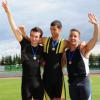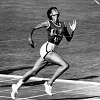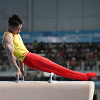#### You may also like### Sport Collection

This is our collection of favourite mathematics and sport materials.### Olympic Starters

Look at some of the results from the Olympic Games in the past. How do you compare if you try some similar activities?### Olympic Turns

This task looks at the different turns involved in different Olympic sports as a way of exploring the mathematics of turns and angles.

# Now and Then

##### Age 7 to 11 Challenge Level:

In $1908$ the Olympic Games were held in London, that's just over $100$ years ago. Then, just after World War $2$ they were again in London in $1948$.

Here are the results from some track events;

$1908$

$100$ metres $10.8$ secs

$200$ metres $22.6$ secs

$400$ metres $50.0$ secs

$800$ metres $112$ secs

$1500$ metres $240$ secs

$1948$

$100$ metres $10.3$ secs

$200$ metres $21.1$ secs

$400$ metres $46.2$ secs

$800$ metres $109$ secs

$1500$ metres $229$ secs

The 2012 London Olympics were another 64 years later.
How did the results differ?
Could you have predicted the results?